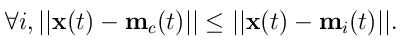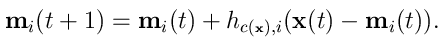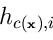# The Self-Organizing Map (SOM)

The SOM is an algorithm used to visualize and interpret large high-dimensional data sets. Typical applications are visualization of process states or financial results by representing the central dependencies within the data on the map.

The map consists of a regular grid of processing units, "neurons". A model of some multidimensional observation, eventually a vector consisting of features, is associated with each unit. The map attempts to represent all the available observations with optimal accuracy using a restricted set of models. At the same time the models become ordered on the grid so that similar models are close to each other and dissimilar models far from each other.

Fitting of the model vectors is usually carried out by a sequential regression process, where t = 1,2,... is the step index: For each sample x(t), first the winner index c (best match) is identified by the conditionAfter that, all model vectors or a subset of them that belong to nodes centered around node c = c(x) are updated asHereis the ``neighborhood function'', a decreasing function of the distance between the ith and cth nodes on the map grid. This regression is usually reiterated over the available samples.

Back to SOM research pagehttp://www.cis.hut.fi/research/som-research/som.shtml